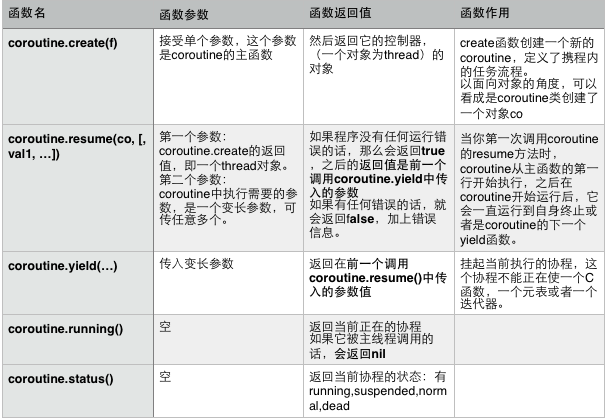用 Lua 的协程 coroutine 控制 Codea 屏幕刷新速度

概述

Codea 中, 函数 draw() 缺省每秒执行 60 次, 我们希望能修改一下它的刷新速度, 于是想到了 Lua 的一个特性:协程 coroutine, 希望试着用它来控制程序执行的节奏, 不过目前对于协程还不太了解, 那就一边看教程, 一边试验好了.

Codea 运行机制

• setup() 只在程序启动时执行一次
• draw() 在程序执行完 setup() 后反复循环执行, 每秒执行 60
• touched()draw() 类似, 也是反复循环执行

setup()

while true do
...
draw()
touched(touch)
...
end

协程 coroutine 的简单介绍

Lua 所支持的协程全称被称作协同式多线程collaborative multithreading）。Lua为每个 coroutine 提供一个独立的运行线路。然而和多线程不同的地方就是，coroutine 只有在显式调用 yield 函数后才被挂起，再调用 resume 函数后恢复运行, 同一时间内只有一个协程正在运行。

Lua 将它的协程函数都放进了 coroutine 这个表里，其中主要的函数如下:协程 coroutine 的使用示例

新建协程 coroutine.create()

-- 先定义一个函数 f
function f ()
print(os.time())
end

-- 为这个函数新建一个协程
co = coroutine.create(f)

• 为一个函数新建协程的意义就在于我们可以通过协程来调用函数.

协程状态 coroutine.status()

> coroutine.status(co)
suspended
>

恢复协程 coroutine.resume()

> coroutine.resume(co)
1465905122
true
>

> coroutine.status(co)
>

挂起协程 coroutine.yield()

function f ()
local k = 0
for i=1,10000000 do
k = k + i
print(i)
coroutine.yield()
end
end

> co = coroutine.create(f)
> coroutine.status(co)
suspended
> coroutine.resume(co)                                                                                                                                                     2
true
> coroutine.status(co)
suspended
> coroutine.resume(co)
3
true
> coroutine.status(co)
suspended
> coroutine.resume(co)
4
true
>

综合使用

time = os.time()
timeTick = 1

function f ()
local k = 0
for i=1,10000000 do
k = k + i
print(i)
-- 如果运行时间超过 1 秒, 则暂停
if (os.time() - time >= timeTick) then
time = os.time()
coroutine.yield()
end
end
end

co = coroutine.create(f)
coroutine.status(co)
coroutine.resume(co)

time = os.time()
timeTick = 1

function f ()
local k = 0
for i=1,10000000 do
k = k + i
-- print(i)
-- 如果运行时间超过 timeTick 秒, 则暂停
if (os.time() - time >= timeTick) then
local str = string.format("Calc is %f%%", 100*i/10000000)
print(str)
time = os.time()
coroutine.yield()
end
end
end

co = coroutine.create(f)

function autoResume()
while true do
coroutine.status(co)
coroutine.resume(co)
end
end

autoResume()

time = os.clock()
timeTick = 0.1
print("timeTick is: ".. timeTick)

function f ()
local k = 0
for i=1,10000000 do
k = k + i
-- print(i)
-- 如果运行时间超过 timeTick 秒, 则暂停
if (os.clock() - time >= timeTick) then
local str = string.format("Calc is %f%%", 100*i/10000000)
print(str)
time = os.clock()
coroutine.yield()
end
end
end

co = coroutine.create(f)

function autoResume()
while true do
coroutine.status(co)
coroutine.resume(co)
end
end

autoResume()

Lua 5.3.2  Copyright (C) 1994-2015 Lua.org, PUC-Rio
timeTick is: 0.1
Calc is 0.556250%
Calc is 1.113390%
Calc is 1.671610%
Calc is 2.229500%
Calc is 2.787610%
Calc is 3.344670%
Calc is 3.902120%
Calc is 4.459460%
Calc is 5.017040%
...

协程 coroutine 跟 Codea 代码框架的结合

function setup()
time = os.clock()
timeTick = 1/2
print("timeTick is: ".. timeTick)

co = coroutine.create(f)
end

function draw()
background(0)
autoResume()
sysInfo()
end

function f ()
local k = 0
for i=1,10000000 do
k = k + i
-- print(i)
-- 如果运行时间超过 timeTick 秒, 则暂停
if (os.clock() - time >= timeTick) then
local str = string.format("Calc is %f%%", 100*i/10000000)
--print(str)
time = os.clock()
coroutine.yield()
end
end
end

function autoResume()
coroutine.status(co)
coroutine.resume(co)
end

-- 显示FPS和内存使用情况
function sysInfo()
pushStyle()
-- fill(0,0,0,105)
-- rect(650,740,220,30)
fill(255, 255, 255, 255)
-- 根据 DeltaTime 计算 fps, 根据 collectgarbage("count") 计算内存占用
local fps = math.floor(1/DeltaTime)
local mem = math.floor(collectgarbage("count"))
text("FPS: "..fps.."    Mem："..mem.." KB",650,740)
popStyle()
end

参考

FreeBlues
2016/06/15
130
0

FreeBlues
2016/06/15
68
0
ngx_lua_API 指令详解（五）coroutine.create，coroutine.resume，coroutine.yield 等集合指令介绍

ngx_lua 模块（原理实现） 1、每个worker（工作进程）创建一个Lua VM，worker内所有协程共享VM； 2、将Nginx I/O原语封装后注入 Lua VM，允许Lua代码直接访问； 3、每个外部请求都由一个Lua...

tinywan1227
2017/07/19
0
0

FreeBlues
2016/06/21
106
0

Future模式： 参见http://www.cnblogs.com/zhiranok/archive/2011/03/26/FuturePattern.html 使用future的好处是即利用了异步的并行能力，又保证主逻辑串行执行，保持简单。 2. Lua 协程 si...

follitude
2016/09/17
43
0

PostgreSQL 11.3 locking

rudi

5
0
Mybatis Plus sql注入器

10
1

4
0

8
0

80
0